### Mathematical Preliminaries: The Set of Real Numbers

#### Learning Outcomes:

• Describe the relationship between real numbers and a continuum

### The Set of Real Numbers

The properties of the set of real numbers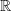is crucial for the study of continuum mechanics. In essence, the set of real numbersis the link between what we call a “number” and the quantity along a continuum. An interested reader should consult with the Wikipedia page on real numbers. The development of the real numbers is a prerequisite for the mathematical description of a continuum. An intuitive illustration for this is to think of the continuous line geometrically as a continuum such that between any two points on the line, there is always another point. Similarly, the real numbers is this representation since for every two real numbers, there is always another real number lying in between. This analogy allows the representation of the line using. The following properties ofare going to be used and extended to linear vector spaces in linear algebra:

The Set of Real Numbers is Closed under Addition:
If two real numbers are added, the result is always another real number. So, we say that the set of real numbers is closed under addition.
The Set of Real Numbers is Closed under Multiplication:
If two real numbers are multiplied, the result is always another real number. So, we say that the set of real numbers is closed under multiplication.
The Set of Real Numbers has a “Zero” Element:
0 is a unique element of the real numbers. It is the neutral element of addition since if any element is added to zero, the result is that element. i.e.,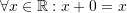Every Element in the Real Numbers has an Inverse Element of Addition:
Indeed, if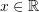, then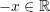, and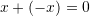.

### Video

1.Mahmood Saleh says:
1.Samer Adeeb says: